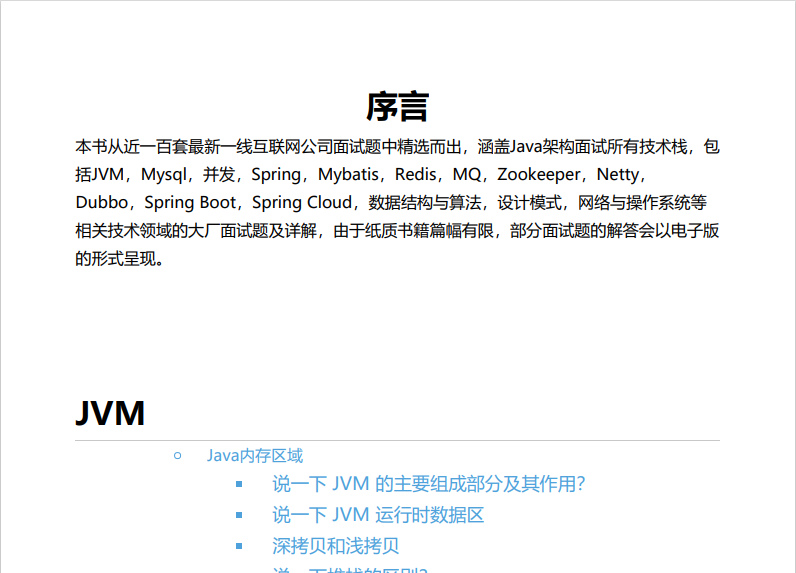# 花 5 分钟手写一个简单的 HashMap，搞定挑剔面试官## 前言HashMap 是 Java 中一中非常常用的数据结构，也基本是面试中的“必考题”。它实现了基于“K-V”形式的键值对的高效存取。JDK1.7 之前，HashMap 是基于数组+链表实现的，1.8 以后，HashMap 的底层实现中加入了红黑树用于提升查找效率。

HashMap 根据存入的键值对中的 key 计算对应的 index，也就是它在数组中的存储位置。当发生哈希冲突时，即不同的 key 计算出了相同的 index，HashMap 就会在对应位置生成链表。当链表的长度超过 8 时，链表就会转化为红黑树。

## 手写 HashMap

### 定义一个 Map 接口

public interface MyMap<K,V> {    V put(K k, V v);    V get(K k);    int size();    V remove(K k);    boolean isEmpty();    void clear();}

### 成员变量

    final static int DEFAULT_CAPACITY = 16;    final static float DEFAULT_LOAD_FACTOR = 0.75f;    int capacity;    float loadFactor;    int size = 0;    Entry<K,V>[] table;

class Entry<K, V>{    K k;    V v;    Entry<K,V> next;    public Entry(K k, V v, Entry<K, V> next){        this.k = k;        this.v = v;        this.next = next;    }}

### 构造方法

public MyHashMap(){    this(DEFAULT_CAPACITY, DEFAULT_LOAD_FACTOR);}public MyHashMap(int capacity, float loadFactor){    this.capacity = upperMinPowerOf2(capacity);    this.loadFactor = loadFactor;    this.table = new Entry[capacity];}

private static int upperMinPowerOf2(int n){    int power = 1;    while(power <= n){        power *= 2;    }    return power;}

### put 方法

@Overridepublic V put(K k, V v) {    // 通过hashcode散列    int index = k.hashCode() % table.length;    Entry<K, V> current = table[index];    // 判断table[index]是否已存在元素    // 是    if(current != null){        // 遍历链表是否有相等key, 有则替换且返回旧值        while(current != null){            if(current.k == k){                V oldValue = current.v;                current.v = v;                return oldValue;            }            current = current.next;        }        // 没有则使用头插法        table[index] = new Entry<K, V>(k, v, table[index]);        size++;        return null;    }    // table[index]为空 直接赋值    table[index] = new Entry<K, V>(k, v, null);    size++;    return null;}

put 方法中，我们通过传入的 K-V 值构建一个 Entry 对象，然后判断它应该被放在数组的那个位置。回想我们之前的论断：

### get 方法

@Overridepublic V get(K k) {    int index = k.hashCode() % table.length;    Entry<K, V> current = table[index];    // 遍历链表    while(current != null){        if(current.k == k){            return current.v;        }        current = current.next;    }    return null;}

### remove 方法

@Overridepublic V remove(K k) {    int index = k.hashCode() % table.length;    Entry<K, V> current = table[index];    // 如果直接匹配第一个节点    if(current.k == k){        table[index] = null;        size--;        return current.v;    }    // 在链表中删除节点    while(current.next != null){        if(current.next.k == k){            V oldValue = current.next.v;            current.next = current.next.next;            size--;            return oldValue;        }        current = current.next;    }    return null;}

current.next = current.next.next;

current 代表我们要删除的节点的前驱节点。

## 最后

1. 扩容问题。在 HashMap 中，当存储的元素数量超过阈值（threshold = capacity * loadFactor）时，HashMap 就会发生扩容（resize）,然后将内部的所有元素进行 rehash，使 hash 冲突尽可能减少。在我们的 MyHashMap 中，虽然定义了加载因子，但是并没有使用它，capacity 是固定的，虽然由于链表的存在，仍然可以一直存入数据，但是数据量增大时，查询效率将急剧下降。

2. 树化问题（treeify）。我们之前讲过，链表节点数量超过 8 时，为了更高的查询效率，链表将转化为红黑树。但是我们的代码中并没有实现这个功能。

3. null 值的判断。HashMap 中是允许存 null 值的 key 的，key 为 null 时，HashMap 中的 hash()方法会固定返回 0，即 key 为 null 的值固定存在 table处。这个实现起来很简单，不实现的情况下 MyHashMap 中如果存入 null 值会直接报NullPointerException异常。

4. 一些其他问题。

end## 评论